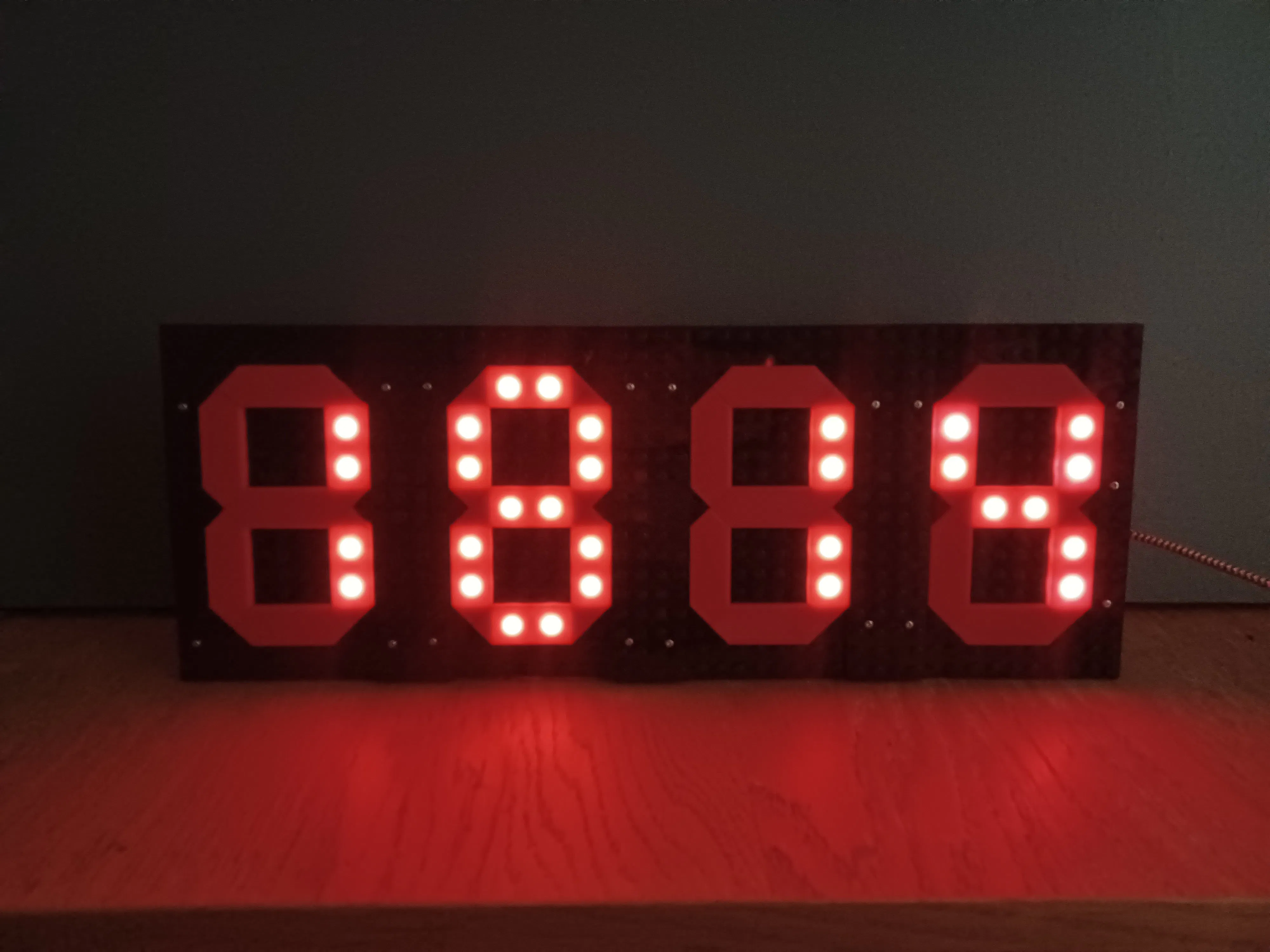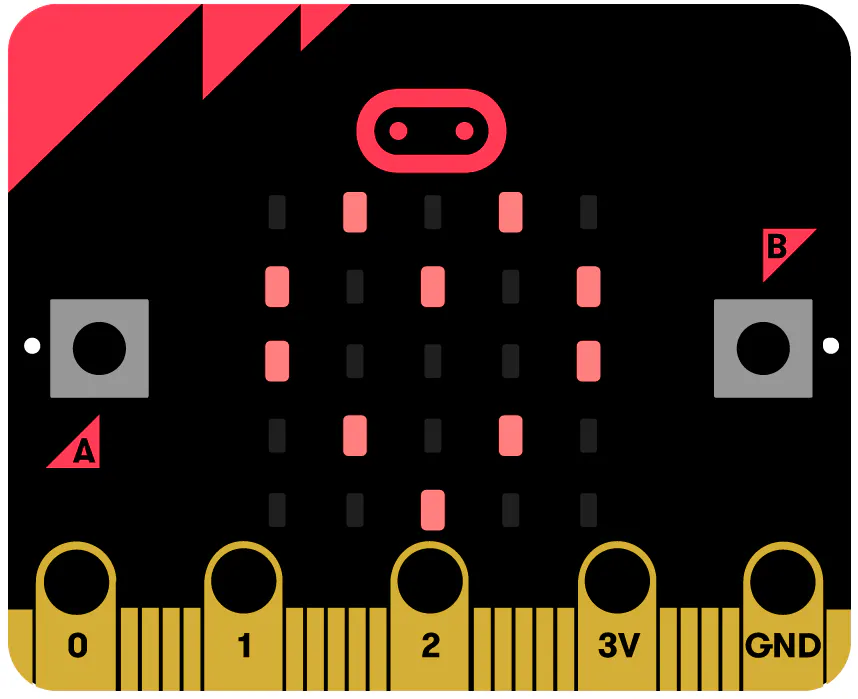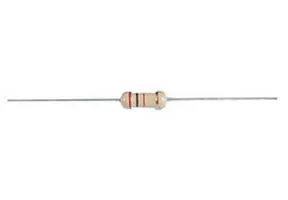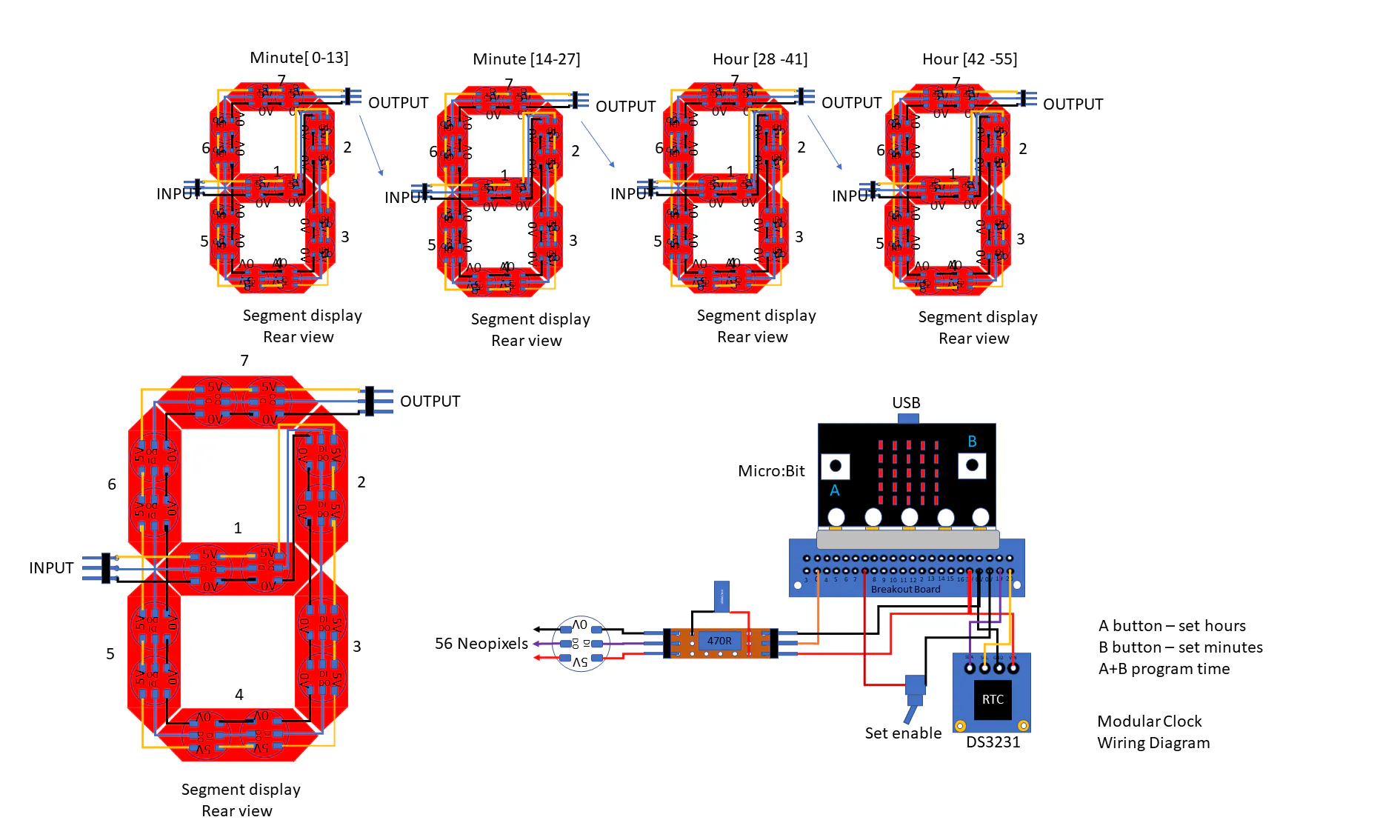# Modular Display Clock

Using custom bricks in conjunction with Neopixels, Microbit and RTC to make modular seven segment display clock.

IntermediateFull instructions provided8 hours6## Things used in this project

### Hardware componentsBBC micro:bit board
×1
×1
 NEOPIXELS
×56
 Edge Connector Breakout
×1
 Switch SPST
×1
 1000uf capacitor
×1Through Hole Resistor, 470 ohm
×1

### Software apps and online servicesMicrosoft MakeCode

### Hand tools and fabrication machines3D Printer (generic)

## Custom parts and enclosures

### tilebase

Support to which the custom segment bricks are attached

### neobase

Support to which the Neoplxels are attached

### Custom tile

Custom segment brick

## Schematics

### MDE schematic

Shows the connections to the individual elements and the arrangement of the Neopixels.## Code

### neolego_clock_v2_py

Python
Makecode Python file
```def set_mode():
global set_enable
if set_enable == 0:
pass
else:
basic.show_string("+")

def on_button_pressed_a():
global set_hr
if set_enable == 1:
set_hr += 1
if set_hr > 23:
set_hr = 0
basic.show_number(set_hr)
input.on_button_pressed(Button.A, on_button_pressed_a)

def pixel_time():
global step, segment_value, digit, inc
step = 3
# Number of 7 segment units
index = 0
while index <= displays - 1:
segment_value = parse_float(time.substr(step, 1))
if index == 3 and msb == 1:
segment_value = 10
digit = segment_list[segment_value]
inc = index * (LED_SEG * 7)
# segment status 1=on, 0=off
for value in digit:
# Number of LED's per segment
for index2 in range(LED_SEG):
if parse_float(value) == 1:
strip.set_pixel_color(inc, neopixel.colors(NeoPixelColors.RED))
else:
strip.set_pixel_color(inc, neopixel.colors(NeoPixelColors.BLACK))
inc += 1
strip.show()
step += -1
index += 1
basic.pause(1000)
def settime():
DS3231.date_time(2020, 2, 15, 4, set_hr, set_min, 0)
basic.show_number(set_hr)
basic.pause(500)
basic.show_number(set_min)
basic.pause(500)

def on_button_pressed_ab():
if set_enable == 1:
settime()
input.on_button_pressed(Button.AB, on_button_pressed_ab)

def Time_split(num: number, num2: number):
global msb, hr_time, min_time, time
msb = 0
basic.show_number(num)
basic.pause(500)
basic.show_number(num2)
basic.pause(500)
if num < 10:
msb = 1
hr_time = "0" + convert_to_text(num)
else:
hr_time = convert_to_text(num)
if num2 < 10:
min_time = "0" + convert_to_text(num2)
else:
min_time = convert_to_text(num2)
time = "" + hr_time + min_time

def on_button_pressed_b():
global set_min
if set_enable == 1:
set_min += 1
if set_min > 59:
set_min = 0
basic.show_number(set_min)
input.on_button_pressed(Button.B, on_button_pressed_b)

inc = 0
digit = ""
msb = 0
segment_value = 0
step = 0
set_min = 0
set_hr = 0
set_enable = 0
time = ""
min_time = ""
hr_time = ""
LED_SEG = 0
displays = 0
segment_list: List[str] = []
strip: neopixel.Strip = None
strip = neopixel.create(DigitalPin.P0, 56, NeoPixelMode.RGB)
strip.clear()
strip.show()
basic.show_string("lclk")
basic.pause(1000)
# Segment assignments for numbers 0 to 9
segment_list = ["0111111",
"0000110",
"1011011",
"1001111",
"1100110",
"1101101",
"1111101",
"0000111",
"1111111",
"1101111",
"0000000"]
# Number of 7 segment displays
displays = 4
# Number of LED's per segment
LED_SEG = 2
hr_time = ""
min_time = ""
time = ""
set_enable = 0
set_hr = 0
set_min = 0

def on_forever():
set_mode()
if set_enable == 0:
Time_split(DS3231.hour(), DS3231.minute())
pixel_time()
basic.clear_screen()
basic.forever(on_forever)
```

## Credits

### C Forde

5 projects • 1 follower